GMAT Question of the Day - Daily to your Mailbox; hard ones only

 It is currently 13 Oct 2019, 13:30GMAT Club Daily Prep

Thank you for using the timer - this advanced tool can estimate your performance and suggest more practice questions. We have subscribed you to Daily Prep Questions via email.

Customized
for You

we will pick new questions that match your level based on your Timer History

Track

every week, we’ll send you an estimated GMAT score based on your performance

Practice
Pays

we will pick new questions that match your level based on your Timer History

Not interested in getting valuable practice questions and articles delivered to your email? No problem, unsubscribe here.The height of an equilateral triangle is the side of a smaller equilat

 new topic post reply Question banks Downloads My Bookmarks Reviews Important topics
Author Message
TAGS:

Hide Tags

Math ExpertV
Joined: 02 Sep 2009
Posts: 58335
The height of an equilateral triangle is the side of a smaller equilat  [#permalink]

Show Tags

2
1100:00

Difficulty:55% (hard)

Question Stats:65% (02:27) correct35% (02:14) wrongbased on 274 sessions

HideShow timer StatisticsThe height of an equilateral triangle is the side of a smaller equilateral triangle, as shown above. If the side of the large equilateral triangle is 1, what is AB?

A. 1 - √3/2
B. 0.25
C. 2 - √3
D. 1/3
E. 1 - √3/4

Attachment:T6036.png [ 7.15 KiB | Viewed 10171 times ]

_________________
CEOV
Joined: 12 Sep 2015
Posts: 3990
Re: The height of an equilateral triangle is the side of a smaller equilat  [#permalink]

Show Tags

8
Top Contributor
3
Bunuel wrote:The height of an equilateral triangle is the side of a smaller equilateral triangle, as shown above. If the side of the large equilateral triangle is 1, what is AB?

A. 1 - √3/2
B. 0.25
C. 2 - √3
D. 1/3
E. 1 - √3/4

Attachment:
T6036.png

The length of one side of the large equilateral triangle is 1...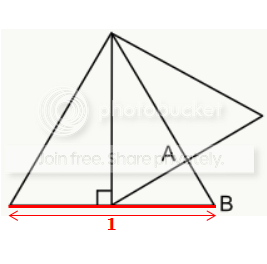The line representing the height of that triangle will cut that side in half, which means the one side (indicated below) will have length 1/2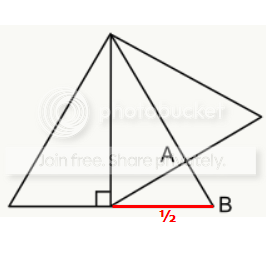Next, since the smaller triangle (in blue below) is also an equilateral triangle, each angle in that triangle is 60 degrees.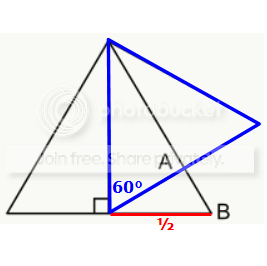This means the angle adjacent to the 60 degree angle must be 30 degrees....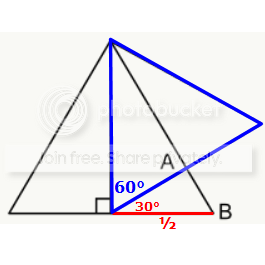Also, since the bigger triangle (in red below) is an equilateral triangle, each angle in that triangle is 60 degrees.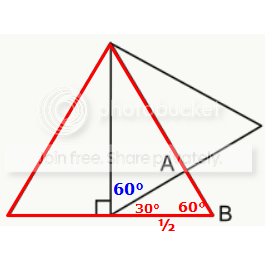Now let's focus our attention on the small red triangle (below)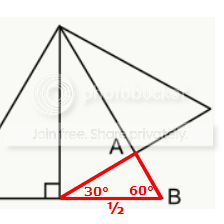Since we know 2 of the angles in the triangle, we can see that the 3rd angle is 90 degrees.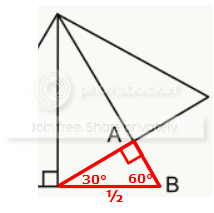This small red triangle is a SPECIAL 30-60-90 triangle, and when we compare it to our "base" 30-60-90 triangle (in blue below), we see that the side opposite the 90-degree angle has length 2 in the base triangle, and the same corresponding side in the red triangle has length 1/2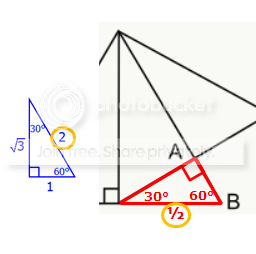This tells us that the red triangle is 1/4 the size of the blue base triangle.
So, since the side opposite the 30-degree angle has length 1 in the base triangle, and the length of the corresponding side in the red triangle = 1(1/4) = 1/4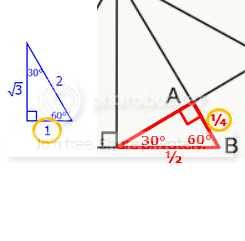RELATED VIDEOS

_________________
General Discussion
Retired ModeratorG
Joined: 26 Nov 2012
Posts: 580
The height of an equilateral triangle is the side of a smaller equilat  [#permalink]

Show Tags

1
Bunuel wrote:The height of an equilateral triangle is the side of a smaller equilateral triangle, as shown above. If the side of the large equilateral triangle is 1, what is AB?

A. 1 - √3/2
B. 0.25
C. 2 - √3
D. 1/3
E. 1 - √3/4

Attachment:
T6036.png

Height of equilateral triangle is √3/2(a)

Given side of larger triangle is a = 1 then height of larger triangle then height is √3/2(1) = √3/2 and this is side of smaller equilateral triangle.

We have side of smaller triangle and height of smaller triangle is √3/2(a) => √3/2(√3/2) = 3/4.

We have height of smaller triangle.

AB is on larger equilateral triangle and we have side of larger triangle and height of smaller triangle to get AB

AB = 1-3/4 = 1/4 = 0.25.

IMO option B.
InternJoined: 17 Mar 2013
Posts: 6
Re: The height of an equilateral triangle is the side of a smaller equilat  [#permalink]

Show Tags

2
the height of the equilateral triangle is also perpendicular bisector for the larger equilateral triangle. So it cuts the side into half. so its 0.5. smaller triangle is equilateral so one of the angle is 60. the other angle would be 180-(90+60) = 30. smaller triangle ABX is 30-60-90 triangle so the side AB will be 0.25.
ManagerB
Joined: 24 Aug 2016
Posts: 61
Location: India
WE: Information Technology (Computer Software)
Re: The height of an equilateral triangle is the side of a smaller equilat  [#permalink]

Show Tags

If the length of the side of equilateral triangle is a, its height will be $$( \sqrt{3} / 2 ) * a$$

So height of bigger triangle using above formula = $$( \sqrt{3} / 2 ) * 1 = ( \sqrt{3} / 2 )$$

The height of bigger triangle = Length of side of smaller triangle = $$( \sqrt{3} / 2 )$$

Height of Smaller triangle = $$( \sqrt{3} / 2 ) * ( \sqrt{3} / 2 ) = 3/4 = 0.75$$

AB = Length of bigger triangle - Height of slammer triangle = 1 -0.75 = 0.25 (B).
_________________
"If we hit that bullseye, the rest of the dominos will fall like a house of cards. Checkmate."
GMATH TeacherP
Status: GMATH founder
Joined: 12 Oct 2010
Posts: 935
Re: The height of an equilateral triangle is the side of a smaller equilat  [#permalink]

Show Tags

Bunuel wrote:The height of an equilateral triangle is the side of a smaller equilateral triangle, as shown above. If the side of the large equilateral triangle is 1, what is AB?

A. 1 - √3/2
B. 0.25
C. 2 - √3
D. 1/3
E. 1 - √3/4

(The formula below we suggest you memorize. It will be used here twice.)

$${h_{eq}} = {{L\sqrt 3 } \over 2}\,\,\,\,\,\left( * \right)\,\,\,\,\,\,\,\,\left( {{\rm{height}}\,\,{\rm{of}}\,\,{\rm{an}}\,\,{\rm{equilateral}}\,\,{\rm{triangle}}\,\,{\rm{with}}\,\,{\rm{side}}\,\,L} \right)$$$$? = AB = {L_{\,{\rm{large}}}} - {h_{{\rm{eq}}\,{\rm{small}}}}\,\, = \,\,\,1 - \,\,{?_{{\rm{temporary}}}}$$

$$\Delta \,{\rm{small}}\,\,:\,\,\,\,\,{L_{\,{\rm{small}}}} = {h_{\,{\rm{eq}}\,{\rm{large}}}}\,\,\mathop = \limits^{\left( * \right)} \,\,\,\,{{1 \cdot \sqrt 3 } \over 2}\,\,\,\,\,\,\,\,\,\, \Rightarrow \,\,\,\,\,\,\,{?_{{\rm{temporary}}}} = {h_{{\rm{eq}}\,{\rm{small}}}}\,\,\,\mathop = \limits^{\left( * \right)} \,\,\,{{\sqrt 3 } \over 2} \cdot {{\sqrt 3 } \over 2} = {3 \over 4}$$

$$? = 1 - {3 \over 4} = {1 \over 4}$$

This solution follows the notations and rationale taught in the GMATH method.

Regards,
Fabio.
_________________
Fabio Skilnik :: GMATH method creator (Math for the GMAT)
Our high-level "quant" preparation starts here: https://gmath.netRe: The height of an equilateral triangle is the side of a smaller equilat   [#permalink] 15 Oct 2018, 11:09
Display posts from previous: Sort by

The height of an equilateral triangle is the side of a smaller equilat

 new topic post reply Question banks Downloads My Bookmarks Reviews Important topics

 Powered by phpBB © phpBB Group | Emoji artwork provided by EmojiOne